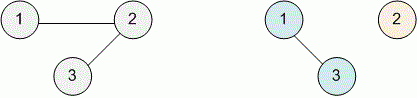Competitions

# Connectivity

Check whether the given undirected graph is connected. That its possible to go from any vertex to any other along the edges of this graph.

#### Input

The first line contains the numbers n and m are separated by spaces - the number of vertices in the graph, respectively (1n100, 1m10000). The following m lines contain two numbers ui and vi by a space (1ui, vin); each such line means that the graph there is an edge between vertices ui and vi.

#### Output

Print "YES", if the graph is connected, and "NO" otherwise.Time limit 1 second
Memory limit 128 MiB
Input example #1
3 2
1 2
3 2

Output example #1
YES

Input example #2
3 1
1 3

Output example #2
NO